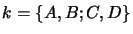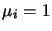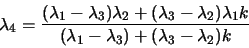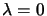Next: Cross Ratios of Pencils Up: Cross-Ratios on the Projective Previous: Cross Ratios and Length

#### Cross Ratios and Projective Bases:

In section 2.1 we saw that any 3 distinct points on the projective line can be used as a projective basis for it. (NB: if we also specify their homogeneous scales, only two are needed, as with M and N above). The cross ratioof any fourth point D with the points of a projective basis A, B, Cdefines D uniquely with respect to the basis. Rescaling toas above, we have(3.2)

As this is invariant under projective transformations, the cross ratio can be used to invariantly position a point with respect to a projective basis on a line. A direct application is reconstruction of points on a 3D line using measured image cross ratios.

Exercise 3.4   : In an image, we see three equally spaced trees in a line. Explain how the position of a fourth tree in the sequence can be predicted. If the first tree lies aton the image line, the second lies at 1and the third at c, what is the image coordinate of the fourth tree?Next: Cross Ratios of Pencils Up: Cross-Ratios on the Projective Previous: Cross Ratios and Length
Bill Triggs
1998-11-13